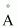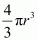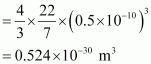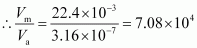# One mole of an ideal gas at standard temperature and pressure occupies 22.4 L (molar volume). What is the ratio of molar volume to the atomic volume of a mole of hydrogen ? (Take the size of hydrogen molecule to be about 1 Å). Why is this ratio so large ?

##### One mole of an ideal gas at standard temperature and pressure occupies 22.4 L (molar volume). What is the ratio of molar volume to the atomic volume of a mole of hydrogen ? (Take the size of hydrogen molecule to be about 1 Å). Why is this ratio so large ?

10 years ago

Radius of hydrogen atom, r = 0.5= 0.5 × 10–10 m

Volume of hydrogen atom =Now, 1 mole of hydrogen contains 6.023 × 1023 hydrogen atoms.

∴ Volume of 1 mole of hydrogen atoms, Va = 6.023 × 1023 × 0.524 × 10–30

= 3.16 × 10–7 m3

Molar volume of 1 mole of hydrogen atoms at STP,

Vm = 22.4 L = 22.4 × 10–3 m3Hence, the molar volume is 7.08 × 104 times higher than the atomic volume. For this reason, the inter-atomic separation in hydrogen gas is much larger than the size of a hydrogen atom.